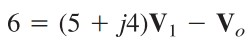omG8HI9LSKFW6lhCj8prTs0Z6lUhdOT9Jhi1Sf4m

# Op Amp AC Circuits

Op Amp can also be analyzed when it is connected with ac sources. The main idea is still the same with the other techniques, such as :
1. Transform the circuit to the phasor or frequency domain.
2. Solve the problem using circuit techniques
3. Transform the resulting phasor to the time domain.

## Op Amp AC Circuits

Please remember, we will use ideal op amps here to ease the explanation. Take notice on the key of analyzing op amp circuits those are :
1. No current enters either of its input terminals
2. The voltage across its input terminals is zero
Let us review the examples below :
1. Determine vo(t) for the op amp in Figure.(1a) if vs = 3 cos 1000t V.Figure 1. For example 1 : (a) the original circuit in the time domain, (b) its frequency domain
Solution :
We first transform the circuit to the frequency domain, as drawn in Figure.(1b), where Vs = 3∠0oω = 1000 rad/s. Applying KCL at node 1 we get
or(1.1)
At node 2, KCL gives(1.2)
Substituting Equations.(1.2) to (1.1) gives
Thus

2. Compute the closed-loop gain and phase shift for the circuit in Figure.(2). Assume that R1 = R2 = 10 kΩ, C1 = 2 uF, C= 1 uF, and ω = 200 rad/s.Figure 2
Solution :
The feedback and input impedances are calculated as
Since the circuit in Figure.(2) is an inverting amplifier, the closed-loop gain is given by
Substituting the given values of R1, R2, C1, C2, and ω, we get
Hence, the closed-loop gain is 0.434 and the phase shift is 130.6o

Untuk Bahasa Indonesia baca Rangkaian AC Op Amp
Related Posts
SHARE